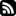Posts Downloads Tags About# Do We Need If?

Introduction to the Scheme programming language specification starts with a wonderful advice: "Programming languages should be designed not by piling feature on top of feature, but by removing the weaknesses and restrictions that make additional features appear necessary." Scheme is a good example of language based on this approach. There is a fairly small set of primitive language constructs out of which the more complex ones are derived.

In the previous post it turned out that boolean values can be encoded as functions for example by doing:

```(define (true a b) a)
(define (false a b) b)
```

Wait a moment. `if` is one of the primitive operations in Scheme. We could make it a library function!

```(define (T a b) a)
(define (F a b) b)
(define (not a) (a F T))
(define (or a b) (a a b))
(define (and a b) (a b b))
(define (if test then else) (test then else))
(if (or F (not (and T F))) 11 22)
;; -> 11

```

It's not quite that simple, though. Scheme, like most other programming languages, uses strict evaluation order. The arguments of a function are evaluated before the function is called. This is in contrast to lazy evaluation, where arguments are evaluated if and when needed. This poses a problem. Both of branches of `if` expressions end up being evaluated. This usually leads to errors, non-termination or undesired side-effects.

Like most strict programming languages, Scheme does not evaluate the body of a lambda expression before it is applied. It evaluates terms to weak head normal form. One can therefore wrap any expression to a lambda expression of no arguments, and apply it when the evaluation should occur. Such functions of no arguments are called thunks.

So, if we use lambda expression representations of booleans, and have `if` handle thunk generation and calling accordingly, then we should have a working `if`. It can no longer be a function, but this is an easy task for a macro.

```(define (T a b) a)
(define (F a b) b)
(define-syntax if
(syntax-rules ()
((if test then)
(if test then F))
((if test then else)
((test (lambda () then) (lambda () else))))))
(if T 11 (car 42))
;; 11, no crash
```

So, at least in theory, we don't need `if`.

Posted: 28.9.2020 by aoh